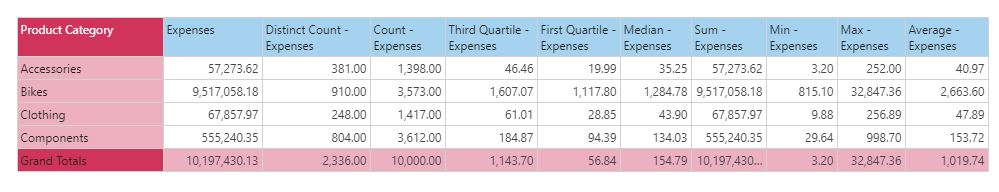#DistinctCount (PQL)

Executes distinct count aggregation on a column of data from the model. The column does NOT need to have numerical data for it to be functional.

• Returned Output: Numeric
• Library: PQL \ Granular \ Aggregate
• Version: 2018.00.000
• Compatibility: Pyramid Query Language (PQL) data sources

#### Syntax

DistinctCount( <Measure> )

* Click on the function's arguments above for more details on the input values.

• The "measure" column needs to be the name of the column in the model [table].[column name] or it can be an existing measure in the model [measures].[column name].
• For details on how to employ and use this function see the granular calculation overview.
##### Different Function types
• This function is like the SQL distinct count function; calculating the distinct set of items in a column of data in your query or model .
• If you wish to find the count for the elements in a specified SET or LIST from a hierarchy, use this semantic function. All counts are always distinct.

#### Examples

To create a new measure that is the distinct count of customer names in the model, using the sample demo model:

DistinctCount( [data].[customerName] )

To create a new measure that is the distinct count of expense values in the model, using the sample demo model:

DistinctCount( [measures].[data Expenses] )

The mechanics of this function are shown below. This grid says that the distinct count of expenses across all 'Accessory' transactions is 381 (vs the simple count of 1398). Which simply means that counting the rows that are in the accessory category in the underlying table (transactions) is 381 after removing duplicates. This produces a different result to the DistinctCount([LIST])function which would simply count the distinct number of elements in the list of items presented instead.